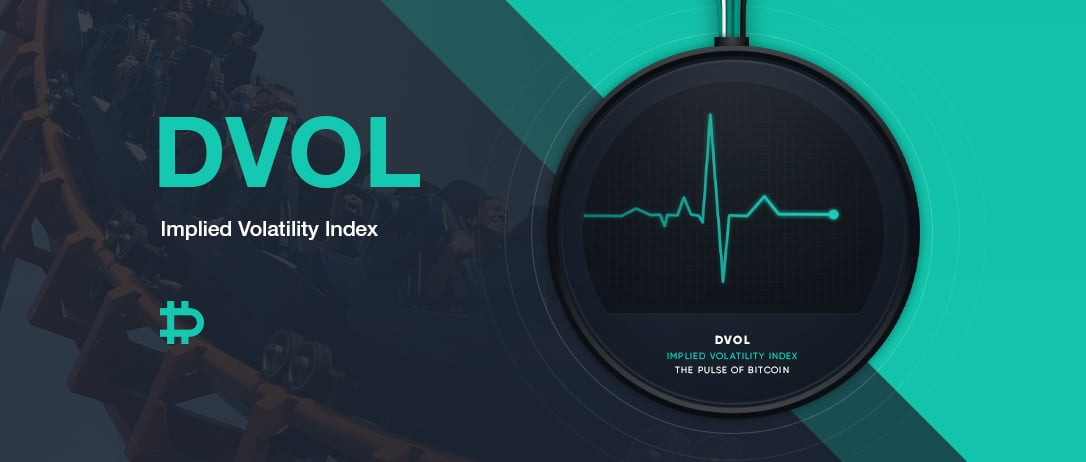Simple exposure to pure implied volatility. This comes in the form of tradable futures on a volatility index – a common instrument in the traditional markets. Deribit is making the first step towards this with the launch of its very own Bitcoin Volatility Index, DVOL, with the purpose to launch futures on this index soon. This index uses the implied volatility smile of the relevant expiries to output one number that gives a gauge of the 30 day annualised implied volatility.

## What does the volatility index measure?

Whilst in traditional market’s, the volatility index is known as a “fear gauge”, when looking at implied volatility in Bitcoin, we instead refer to this volatility index as an “action gauge” or a “fear and greed gauge”. Unlike the majority of traditional markets, Bitcoin options often have positive skew and the large moves are often expected on the upside as well as downside.

## How to trade volatility?

The implied volatility of Bitcoin options gives an indication of the expected movement of Bitcoin. Options are a necessary piece of information to calculate a volatility index. Nearly 9 out of 10 times that a Bitcoin option is traded in the world, it is traded on Deribit. This makes it essential that Deribit options are used to calculate a meaningful volatility index for Bitcoin. Trading options to trade volatility (by trading Vega) comes entangled with other components such as Delta (exposure to movement in the underlying) and Theta (exposure to time decay) amongst others. Volatility Index Futures are not only a very effective and simple method to trade volatility but also opens up new trading strategies such as more possibilities of realised vs implied volatility strategies, hedging volatility exposure from options, volatility mean reversion and momentum strategies.

## How to interpret DVOL?

The DVOL is forward-looking volatility. What does the calculated value mean? It gives the 30 day (forward-looking) annualised expectation of volatility. To get a rough idea of the expected daily move in Bitcoin, just divide this value by 20. For example, DVOL = 90 gives an expected daily move of 4.5% (More precisely, you should divide DVOL by the square root of 365 to get an estimate of the expected daily move.)

## Methodology

The outline for the calculation of the Bitcoin Volatility Index is the following: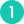Select the 2 expiries, closest to 30 days out, on either side of the 30 days.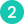Calculate the option price using the market depth of bids and asks. If the bid/ask spread is too wide then fallback to using trade prices during the last minute and if that is not available then use the mark price from 1 min ago.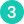Calculate the option implied synthetic. If not enough info/options not tight enough to calculate this well, then use the mark price of the synthetic from 1 min ago.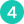Discard the ITM calls and puts. Discard options with very low premiums.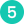Use the variance swap methodology to calculate the variance for the near term and longer-term expiries. Interpolate between the 2 expiries and take the square root.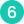Filter noise and smooth out value calculated, resulting in the index calculation.

## Crunching the numbers

Select Expiry1 to be the furthest expiry that has less than or equal to 30 days to expiry, which is also older than 1 hour since it was introduced. Select Expiry2 to be the closest expiry that has less than or equal to 30 days to expiry which is also older than 1 hour since it was introduced. We ignore expiries (and instruments) that are newly introduced, with time since introduction to be less than 1 hour [Parameter: IgnoreNewInstrument]. This is to allow the expiry/instruments to settle since bids and asks will be wider and hence may not reflect the true volatility initially. For each expiry, calculate the “Depth Bid” and “Depth Ask” which is so that we take the depth and volumes into account. We define this in the following manner.

Note: All option prices used are BTC prices and not USD.

## Using the Market Depth

Remove 0.5 volume [Parameter: RemoveVolume] from only the top level of the depth. If there is only 0.5 or less volume on the top level, then the next level is taken as the first level. Use a total of 5 levels [Parameter: DepthLevels], including the first level. This is done by using the price of the first level and creating 4 additional levels with each level created 1 tick behind the previous level. The volume from the market is then matched to those price levels (minus the 0.5 volume if the first original level was not removed), until a cumulative size of 10 BTC [Parameter: DepthVolume] volume is reached. If the total volume on those levels is less than 10 BTC, then 1 further level behind is created with the remaining volume to make it 10 BTC.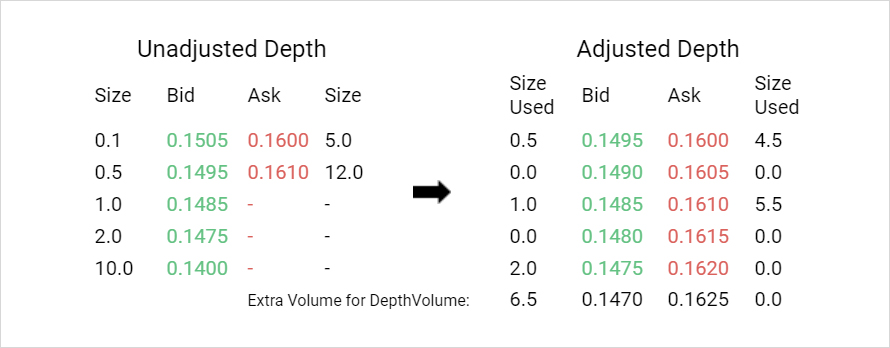Calculate the average volume-weighted price from the transformed depth. So in example above, that would give:

Depth Bid = (0.5×0.1495+0×0.1490+1×0.1485+0×0.1480+2×0.1475+6.5×0.1470)/10 = 0.147375, a similar calculation for the ask side, gives Depth Ask = 0.16055

## Instrument Price

If the spread is not wide and there exists a non-zero Depth Bid and a Depth Ask, then the Instrument Price = Mid Depth Price = (Depth Ask – Depth Bid)/2. What is wide? When Depth Ask – Depth Bid ≥ max(min(MaxSpreadBidRatio * DepthBid, MaxSpreadWidth), MinSpreadWidth) – see end of whitepaper for the default parameter values. If wide, then we use a fallback price as shown below.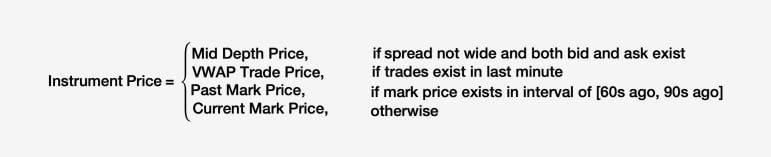The VWAP Trade Price is the volume-weighted average price on all trades that happened on that instrument in the last 1 minute. If there were no trades in the past 1 minute we use a past mark price. We look at the most recent past mark price that is the interval of [60sec ago, 90sec ago]. If for any reason, the past mark price is not available in the interval, then we fall back to the current mark price. If the Instrument Price < 0.002 BTC [Parameter: PriceCutoff], we discard that book from further calculations. Why do we discard very low premium books? The granularity of information held in them with a high tick size relative to Vega means they can provide a distorted picture of the market depth in volatility space.

## Forward Price Calculation

Next, we have to calculate the forward price (synthetic price) for each expiry. We do this using the option implied synthetic where there are at least 2 full strikes that have both the call and put using a Mid Depth price. We calculate abs(Call Mid Depth Price – Put Mid Depth Price) and select the StrikeMin = the strike with the lowest value.If multiple strikes share the lowest value then we calculate the implied forward using each of those strikes and take the average. If there aren’t at least 2 strikes [Parameter: MinFullStrikesForMarketSyn] where both call and put are using the Mid Depth Price, then the forward equals the most recent past synthetic/future mark price in the interval [60s ago, 90s ago] for that expiry. And an added safety, if there is no past synthetic price in that interval, then we use the current synthetic/future price for that expiry. For each expiry, we take the Strike Cutoff to be the strike equal to, or closest strike below the Forward for that expiry. We discard all instruments where the strikes for calls are less than the Expiry Strike Cutoff and puts are greater than the Expiry Strike Cutoff. So there will be 2 instruments at the Strike Cutoff and a maximum of one instrument on the other strikes.

## Calculating Variance

Looking at the filtered list of strikes which contain at least 1 non-discarded instrument, for each strike, calculate the StrikeWidth.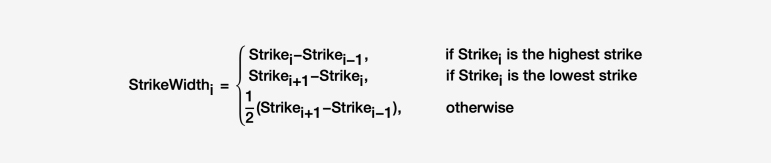At the StrikeCutoff, the StrikePrice is the average of the InstrumentPrice of the call and put. Everywhere else, the StrikePrice is the InstrumentPrice of the non-discard instrument at that strike.Using the strike contributions, we can then calculate the variance of the expiry. t represents the time in years to the maturity of that expiry.Note that we effectively can get the volatility index of any expiry withWe now do a time-weighted interpolation between the 2 selected expiries to calculate a raw value.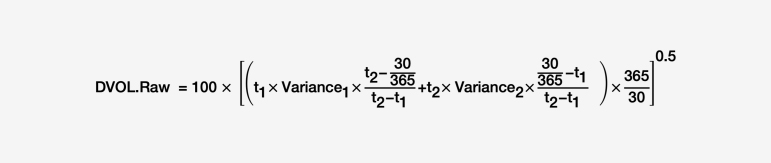DVOL.Raw is calculated every second and finally, we need to filter noise and smoothen DVOL.Raw to get a final value for the index. We do this by taking the interquartile mean of the last 120 points [Parameter: DataPointsForSmoothing] to get the DVOL.IQM every second.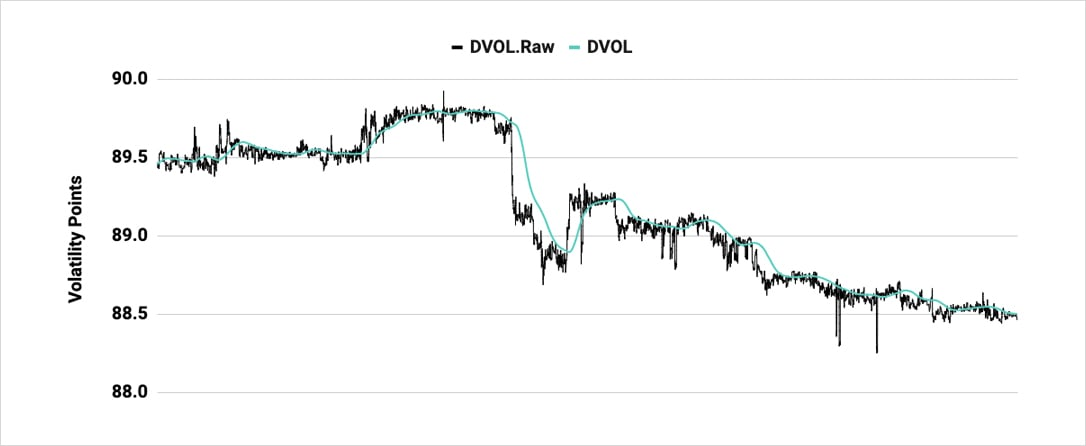Next, we calculate an exponential moving average (EMA) of the last 120 points [Parameter: EMAPeriod] of DVOL.IQM to get the final value for the Bitcoin Volatility Index, DVOL.

The parameters stated in this whitepaper are examples and the defaults. However, these parameters are subject to change by Deribit. Parameter changes will be announced 48 hrs before implementation.

Parameter Value Description
IgnoreNewInstrument 1 hour Does not take any new instruments/expiries that are newer than this into account.
DepthVolume 10 BTC Volume searched for in depth to determine and the price levels used.
DepthLevels 5 Number of levels after the first level is established.
RemoveVolume 0.5 BTC Removed from the top level of the unadjusted depth. If this is greater than or equal to volume on top level, then that level is removed.
FallbackOptionDelay 60 sec Used for the lookback period for the VWAP Trade Price and also used when falling back to Past Mark Price which is the most recent price in the interval [FallBackOptionDelay seconds ago, FallBackOptionDelay + CaptureInterval seconds ago].
MinFullStrikesForMarketSyn 2 Number of full strikes needed with both call and put using Mid Depth Price for the market implied option synthetic to be used.
FallbackSyntheticDelay 60 sec When taking the past mark synthetic price, we look at the most recent mark price in the interval [FallBackOptionDelay seconds ago, FallBackOptionDelay + CaptureInterval seconds ago].
CaptureInterval 30 sec When taking the past mark synthetic price, we look at the most recent mark price in the interval [FallBackOptionDelay seconds ago, FallBackOptionDelay + CaptureInterval seconds ago].
MinSpreadWidth 0.0025 BTC An upper bound to compare the Depth Spread to. Spread is wide if Depth Ask – Depth Bid ≥ max( min(MaxSpreadBidRatio * DepthBid,MaxSpreadWidth), MinSpreadWidth).
MaxSpreadWidth 0.03 BTC An upper bound to compare the Depth Spread to. Spread is wide if Depth Ask – Depth Bid ≥ max( min(MaxSpreadBidRatio * DepthBid,MaxSpreadWidth), MinSpreadWidth).
MaxSpreadBidRatio 12% Multiplied by the DepthBid to compare the spread.
DataPointsForSmoothing 120 Take the IQM using this number of points of DVOL.Raw to calculate DVOL.IQM.
EMAPeriod 120 Takes the exponential moving average, looking back over this number of points.

AUTHOR(S)Shaun Fernando

RECENT ARTICLES